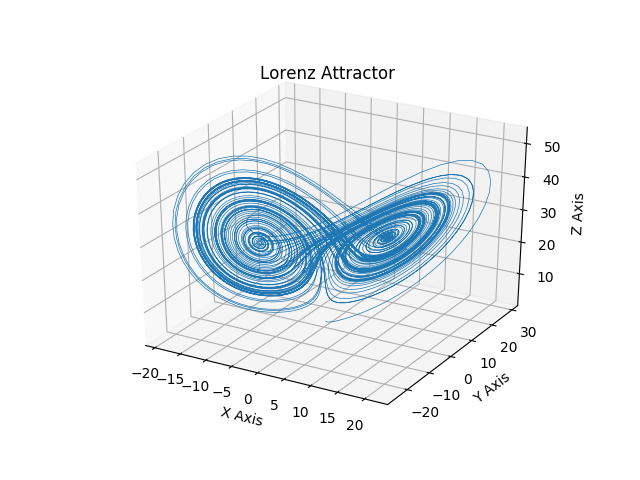# Does the Lorenz attractor contain critical points?

Take a look at a standard picture of the Lorenz attractor (with the classical parameter values):The Lorenz attractor is a complicated fractal subset of $$\mathbb{R}^3$$ of Hausdorff dimension > 2. Does this fractal include the two critical points at the centers of the spirals and/or the one at the origin?

According to what formal definition of attractor?

Let $$\varphi$$ be the flow of the Lorenz system with the classical parameter values. There exists a forward invariant open set $$U$$ (a double torus) containing the origin but not the other two critical points. The Lorenz attractor is $$\mathcal{A} = \bigcap_{t\geqslant 0} \varphi(U,t).$$ Since the origin is an equilibrium point contained in $$U$$, it is also contained in $$\mathcal{A}$$. Since $$U$$ is forward invariant and does not contain the other two critical points, $$\mathcal{A}$$ also does not contain the other two critical points.